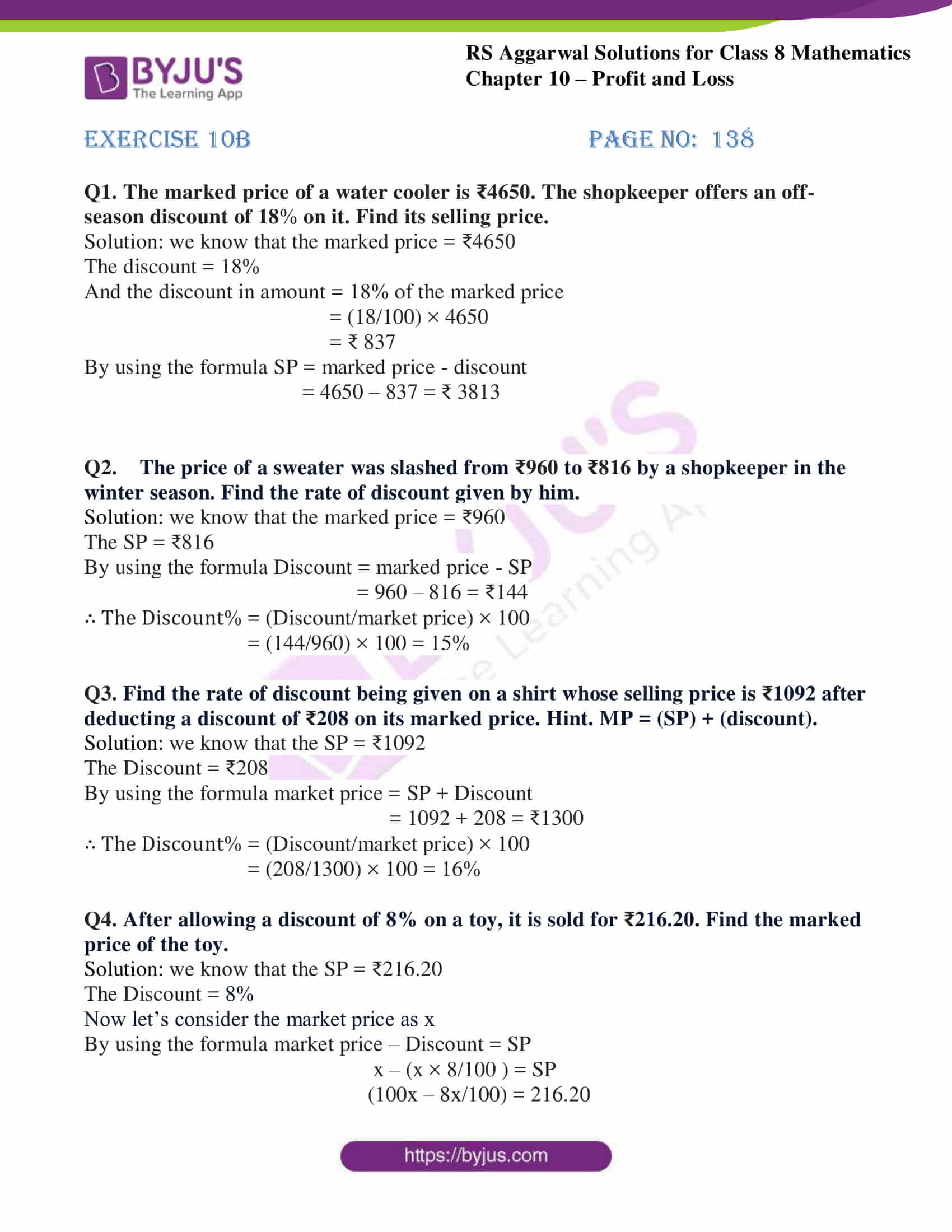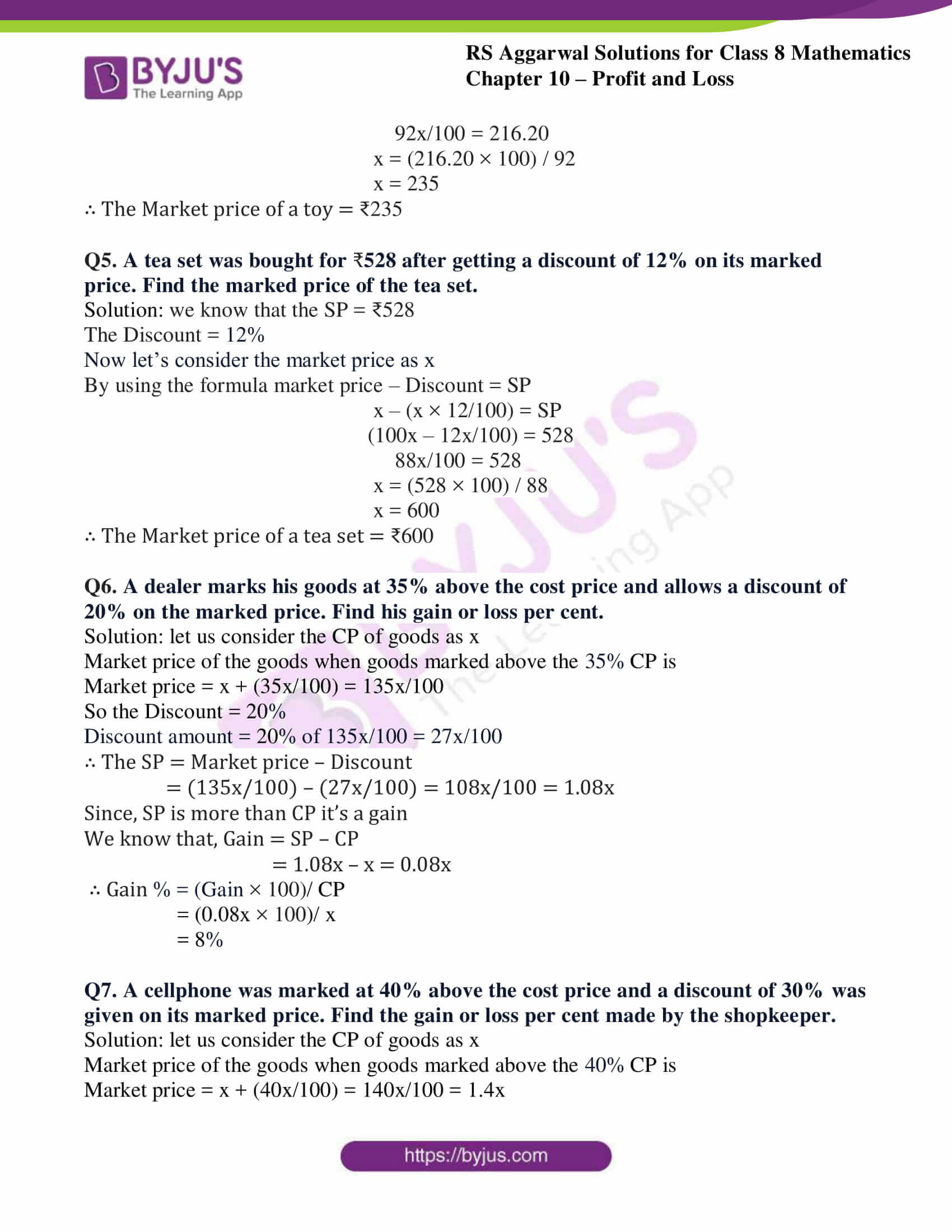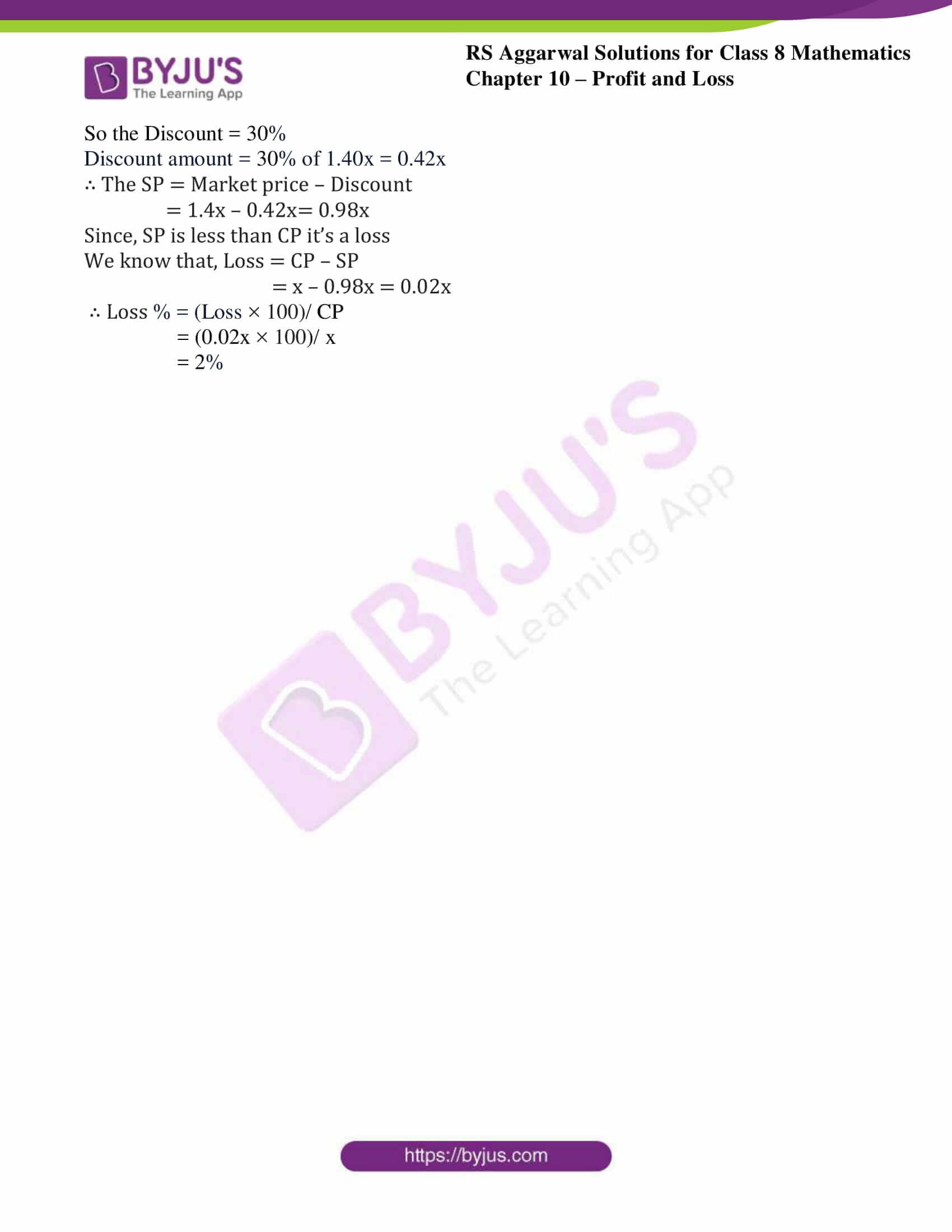# RS Aggarwal Solutions for Class 8 Chapter 10 - Profit and Loss Exercise 10B

Students can refer and download RS Aggarwal Solutions for Class 8 Maths Chapter 10- Exercise 10B, Profit and Loss from the links provided below. Our experts have solved the RS Aggarwal Solutions to ensure that the students are thorough with their basic concepts by practising the solutions. In Exercise 10B of RS Aggarwal Class 8 Maths, we shall see more about discount related questions.

## Download PDF of RS Aggarwal Solutions for Class 8 Maths Chapter 10- Exercise 10B### Access Answers to RS Aggarwal Solutions for Class 8 Maths Chapter 10- Profit and Loss Exercise 10B

Q1. The marked price of a water cooler is ₹4650. The shopkeeper offers an off-season discount of 18% on it. Find its selling price.

Solution: we know that the marked price = ₹4650

The discount = 18%

And the discount in amount = 18% of the marked price

= (18/100) × 4650

= ₹ 837

By using the formula SP = marked price – discount

= 4650 – 837 = ₹ 3813

Q2. The price of a sweater was slashed from ₹960 to ₹816 by a shopkeeper in the winter season. Find the rate of discount given by him.

Solution: we know that the marked price = ₹960

The SP = ₹816

By using the formula Discount = marked price – SP

= 960 – 816 = ₹144

∴ The Discount% = (Discount/market price) × 100

= (144/960) × 100 = 15%

Q3. Find the rate of discount being given on a shirt whose selling price is ₹1092 after deducting a discount of ₹208 on its marked price. Hint. MP = (SP) + (discount).

Solution: we know that the SP = ₹1092

The Discount = ₹208

By using the formula market price = SP + Discount

= 1092 + 208 = ₹1300

∴ The Discount% = (Discount/market price) × 100

= (208/1300) × 100 = 16%

Q4. After allowing a discount of 8% on a toy, it is sold for ₹216.20. Find the marked price of the toy.

Solution: we know that the SP = ₹216.20

The Discount = 8%

Now let’s consider the market price as x

By using the formula market price – Discount = SP

x – (x × 8/100 ) = SP

(100x – 8x/100) = 216.20

92x/100 = 216.20

x = (216.20 × 100) / 92

x = 235

∴ The Market price of a toy = ₹235

Q5. A tea set was bought for 528 after getting a discount of 12% on its marked price. Find the marked price of the tea set.

Solution: we know that the SP = ₹528

The Discount = 12%

Now let’s consider the market price as x

By using the formula market price – Discount = SP

x – (x × 12/100) = SP

(100x – 12x/100) = 528

88x/100 = 528

x = (528 × 100) / 88

x = 600

∴ The Market price of a tea set = ₹600

Q6. A dealer marks his goods at 35% above the cost price and allows a discount of 20% on the marked price. Find his gain or loss per cent.

Solution: let us consider the CP of goods as x

Market price of the goods when goods marked above the 35% CP is

Market price = x + (35x/100) = 135x/100

So the Discount = 20%

Discount amount = 20% of 135x/100 = 27x/100

∴ The SP = Market price – Discount

= (135x/100) – (27x/100) = 108x/100 = 1.08x

Since, SP is more than CP it’s a gain

We know that, Gain = SP – CP

= 1.08x – x = 0.08x

∴ Gain % = (Gain × 100)/ CP

= (0.08x × 100)/ x

= 8%

Q7. A cellphone was marked at 40% above the cost price and a discount of 30% was given on its marked price. Find the gain or loss per cent made by the shopkeeper.

Solution: let us consider the CP of goods as x

Market price of the goods when goods marked above the 40% CP is

Market price = x + (40x/100) = 140x/100 = 1.4x

So the Discount = 30%

Discount amount = 30% of 1.40x = 0.42x

∴ The SP = Market price – Discount

= 1.4x – 0.42x= 0.98x

Since, SP is less than CP it’s a loss

We know that, Loss = CP – SP

= x – 0.98x = 0.02x

∴ Loss % = (Loss × 100)/ CP

= (0.02x × 100)/ x

= 2%

## RS Aggarwal Solutions for Class 8 Maths Chapter 10- Exercise 10B

Exercise 10B of RS Aggarwal Class 8, Profit and Loss. This exercise mainly deals with discount- In order to increase sales or clear the old stock, sometimes the shopkeepers offer a certain percentage of rebate on the marked price. This rebate is known as a discount.

Students are suggested to practice the problems on a regular basis which will help them excel in their exams and increase their overall percentage. Practicing as many times as possible helps in building time management skills and also boosts the confidence level to achieve high marks.